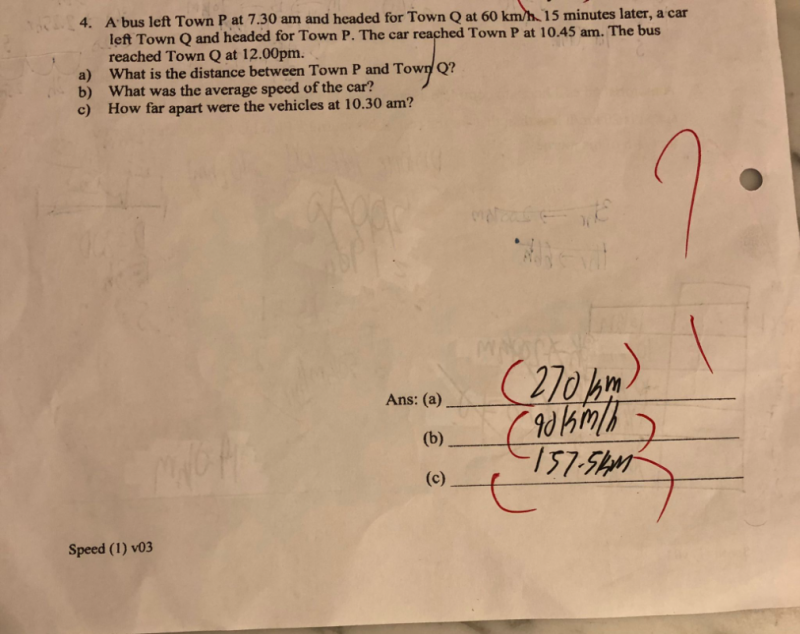# Questiona. Total time taken by the bus = 12 – 7:30 = 4.5 hrs
Distance between P and Q = Speed * Time taken = 60 * 4.5 = 270km

b. Total time taken by the car = 10:45 – 7:45 = 3 hrs
Speed of the car = Distance / Time taken = 270 / 3 = 90 km/h

c. At 10:30am,
Distance of car from Q = Speed * Time taken = 90 * 2:45 = 180 + 45/60 *90 = 247.5 km
Distance of bus from P = Speed * Time taken = 60 * 3 = 180 km
So distance between car and bus =

0 Replies 1 Like ✔Accepted Answer

(a) Bus speed = 60

Bus time 7:30-12:00 = 4.5

Hence distance PQ = 60 x 4.5 = 270km

(b) Car total time 7:45 – 1:45 = 3

Car total distance = 270

Hence Car average speed = 270/3 = 90km/h

(c)  Bus Time  7:30-10:30 = 3

Bus speed = 60

Bus distance travelled = 60 x 3 = 180        Bus remaining distance = 270-180 = 90

Car Time 7:45-10:30 = 2.75

Car speed = 90

Car distance travelled = 90 x 2.75 = 247.5   Car remaining distance = 270 – 247.5 = 22.5

Hence distance between bus and car at 10:30 = 270 – 90 – 22.5 = 157.5km

0 Replies 2 Likes